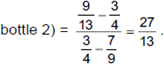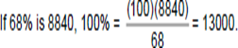# CAT 2017 – Slot 2 – Quantitative Ability - The numbers 1, 2,..., 9 are arranged in a 3 X 3 square grid

Q. 1: The numbers 1, 2,…, 9 are arranged in a 3 X 3 square grid in such a way that each number occurs once and the entries along each column, each row, and each of the two diagonals add up to the same value.
If the top left and the top right entries of the grid are 6 and 2, respectively, then the bottom middle entry is

Explanation:-   The square grid is filled by 9 numbers from 1 to 9. Their sum (1 + 2 + 3 +… 9) equals 45. Since the sum of  numbers in each row and each column and each diagonal must be equal, the sum of terms in each row and in each column and in each diagonal, must be 15. For this to happen, the middle element in the 2nd row and the 2nd column must be the middle-most term of the 9 terms, i.e. 5. The corner elements in the first row are 6 and 2 (given), so the middle element in the first row must be 7. In the 2nd column, the top most element is 7 and the middle element is 5, so the bottom row middle element must be 3.

##### Q. 2: In a 10 km race. A, B, and C, each running at uniform speed, get the gold, silver, and bronze medals, respectively. If A beats B by  1 km and B beats C by 1 km, then by how many metres does A beat C?

Explanation:- A beats B by 1 km, means A travels 10 km in the same time that B travels 9 km. The ratio of speeds of A and B is  10 : 9. Similarly, the ratio of speeds of B and C is 10 : 9.

A : B = 10 : 9 B : C = 10 : 9

⇒ The ratio of speeds of A : B : C = 100 : 90 : 81

In the same time that A travels 100 m, C travels 81 metres

⇒ In the same time that A travels 10000 m, C would travel 8100 m or A would beat C by 1900 m. Ans: (1900)

Q. 3: Bottle 1 contains a mixture of milk and water in 7 : 2 ratio and Bottle 2 contains a mixture of milk and water in 9 : 4 ratio. In what ratio of volumes should the liquids in Bottle 1 and Bottle 2 be combined to obtain a mixture of milk and water in 3 : 1 ratio?
A) 27 : 14
B) 27 : 13
C) 27 : 16
D) 27 : 18

Explanation:- Concentration of milk in the first bottle is 7/9 and that in the second bottle is 9/13. They need to be mixed in a certain ratio to get a solution which has 3/4th milk.
Applying alligation,
(Liquid taken from bottle 1)/(Liquid taken from bottle 2) =Q. 4: Arun drove from home to his hostel at 60 miles per hour. While returning home he drove half way along the same route at a speed of 25 miles per hour and then took a bypass road which increased his driving distance by 5 miles, but allowed him to  drive at 50 miles per hour along this bypass road. If his return journey took 30 minutes more than his onward journey, then the total distance traveled by him is
A) 55 miles
B) 60 miles
C) 65 miles
D) 70 miles

Explanation:- Let the distance from his home to his hostel be x miles. Time taken on his onward journey = x/60 hours

Time taken on his return journey = (x/2)/25 + (x/2 + 5)/50

Given, his return journey took 0.5 hours more than his onward journey

⇒ x/60 + 0.5 = x/50 + (x/2 + 5)/50 Upon solving, x = 30

Therefore, total distance travelled = 30 + 15 + 20 = 65 miles.

Q. 5: Out of the shirts produced in a factory, 15% are defective, while 20% of the rest are sold in the domestic market. If the remaining 8840 shirts are left for export, then the number of shirts produced in the factory is
A) 13600
B) 13000
C) 13400
D) 14000

Explanation:- A total of 15% shirts are defective. Of the remaining 85%, 20% are sold in the domestic market. So, 20% of 85%, i.e. 17% are sold in the domestic market and the remaining 68% are exported. But, it is given that 8840 shirts were exported.##### Checkout Other Questions of CAT 2017 Slot 2 Paper:

Verbal Ability :              |   Q.01- Q.06  |  Q.07- Q.12  |  Q.13- Q.18  |  Q.19- Q.21  |  Q.22- Q.24  |  Q.25- Q.29  |  Q.30 – Q.34  |

Logical Reasoning :    |   Q.29 – Q.32  |

Quantitative Aptitude: |

### Free Material Area